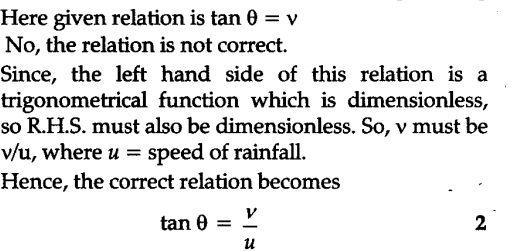# A man walking briskly in rain with speed v must slant his umbrella forward making an angle 0 with the vertical

A man walking briskly in rain with speed v must slant his umbrella forward making an angle 0 with the vertical. A student derives the following relation between $\upsilon$ and $\theta$ : tan $\theta$ = $\upsilon$ and checks that the relation has a correct limit as $\upsilon \to 0,\theta \to 0$, as expected. (We are assuming there is no strong wind and that the rain falls vertically on a stationary man). Do you think this relation can be correct ? If not guess the correct relation